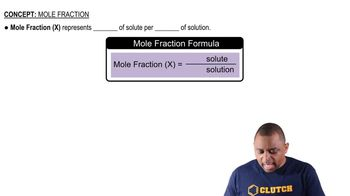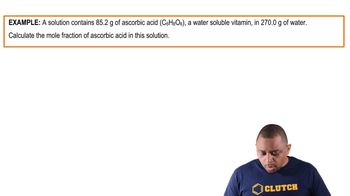Start typing, then use the up and down arrows to select an option from the list.
3:38 minutes
Problem 64a
Textbook Question

# A solution is prepared by dissolving 20.2 mL of methanol (CH3OH) in 100.0 mL of water at 25 °C. The final volume of the solution is 118 mL. The densities of methanol and water at this temperature are 0.782 g>mL and 1.00 g>mL, respectively. For this solution, calculate the concentration in each unit. e. mole percentVerified Solution
This video solution was recommended by our tutors as helpful for the problem above.
47views